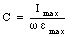## Solutions Homework Set # 9 - Physics 122

Problem 1

When the coil is connected to the oscillator, it forms an RL circuit. The impedance of the RL circuit is equal to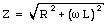The amplitude of the current is equal toThis equation can be rewritten as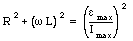or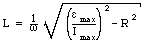Problem 2

We assume that the field produced by the solenoid is equal to that of a perfect solenoid. The field without a core is equal to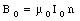The magnetic field present with the core is equal to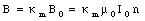The energy density is thus equal to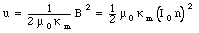Problem 3

The magnetic field inside the solenoid is equal toThis equation can be used to determine the current that must be used to generate the magnetic field B: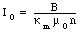Problem 4

Right after the battery is connected, the current in the circuit will be zero. The entire emf of the battery will thus be across the inductors. For conductor 1 we thus conclude that: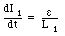Problem 5.

Using the same procedure outline in Problem 4 we conclude that for conductor 2: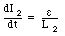Problem 6

In a steady state, the current is constant (independent of time) and the potential drop across the inductors is thus equal to zero. This implies that the entire emf of the battery is across the resistor, and consequently the current through the resistor will be equal to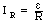Problem 7

The parallel connection of the two inductors requires that they have the same potential difference across them. Thus: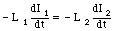This equation requires that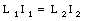The sum of these current must be equal to the current through the resistor. Thus: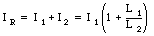The current through inductor 1 is thus equal to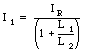Problem 8

The current through inductor 2 is equal to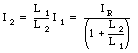Problem 9

The impedance of the circuit is equal to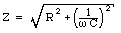Problem 10

The impedance of the circuit is equal to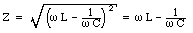The amplitude of the current is equal toProblem 11

Since all components are hooked up in series, the amplitude of the instantaneous current across the inductor will be the same as the amplitude of the instantaneous current across the capacitor. See solution for Problem 10.

Problem 12

The impedance of the LR circuit is equal toThe amplitude of the current is thus equal to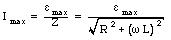Problem 13

By using a phasor diagram (see for example Figure 34.18 in Ohanian) we can conclude that the phase angle [phi] can be determined from the following relation: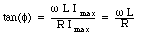Thus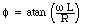Problem 14

The power dissipated in the resistor is equal to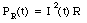The current in the resistor is equal toThe average power dissipated in the resistor is equal to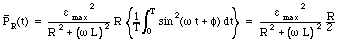Problem 15

NOTE: the graph for this problem can differ for different assignments. This solution is for the graph shown on the assignment of Frank Wolfs.

At time t1 the current has completed fT oscillations, where f = 1.75 for my graph. Thus: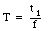The angular frequency of the generator is thus equal to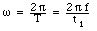Problem 16

The current through the circuit element is equal to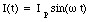The voltage across the circuit element is equal to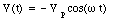The power dissipated is in the circuit element at time t is equal to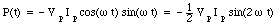The average power dissipated in the circuit element during one period is equal to zero.

Problem 17

Since the voltage and current are out of phase, the circuit elements is not a resistor. Since the current leads the voltage by 90deg., we conclude that the circuit elements must be a capacitor. The amplitude of the current in a circuit with a source of emf and a capacitor is equal to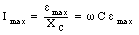The capacitance of the capacitor is thus equal to